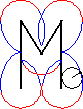[home] [lexicon] [problems] [tests] [courses] [auxiliaries] [notes] [staff]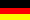Mathematics-Online course: Basic Mathematics - Real Numbers

# Real Numbers

The setof all (finite and infinite) decimal numbers is referred to as real numbers. Real numbers can be identified with the points of the real line. A number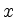corresponds to the distance from the origin, while its algebraic sign indicates whetherbelongs to the positive or negative half line.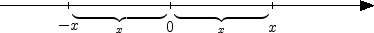The rational numbersare dense in, that is every irrational number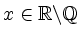can be approximated by fractions to an arbitrary degree of accuracy. Unlikehowever,is not countable.

Together with addition and multiplication, the real numbers form a field. Moreover,is complete, that is every convergent sequence of real numbers has a limit in.

(Authors: Höllig/Kopf/Abele)